#### python里画出“热图”

统计/机器学习 数据可视化 Python    浏览次数：10303        分享

1

python里怎么画“热图”？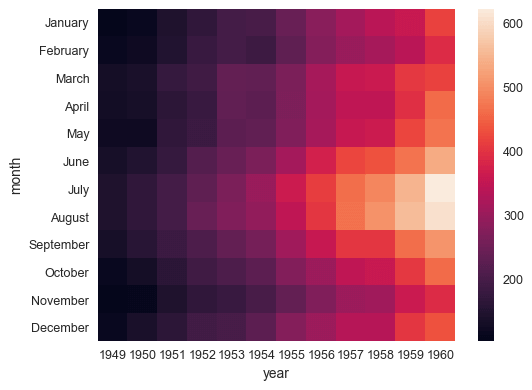Robin峰   2018-01-12 23:46

##### 4个回答
7

import matplotlib.pyplot as plt
import seaborn as sns
import numpy as np

a = np.random.uniform(0, 1, size=(10, 10))
sns.heatmap(a, cmap='Reds')
plt.show()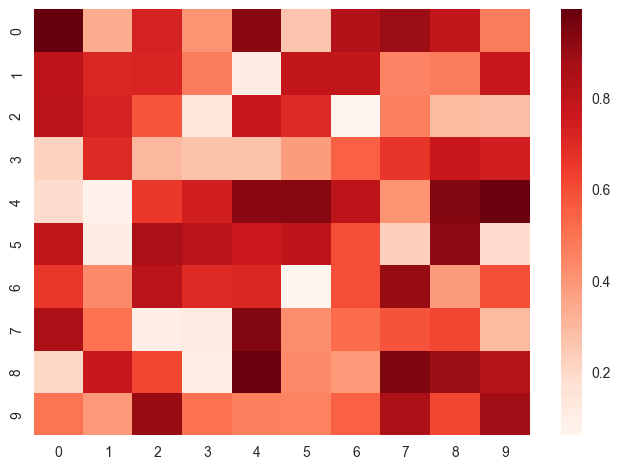a = np.random.uniform(0, 1, size=(10, 10))
sns.heatmap(a, cmap='GnBu')
plt.show()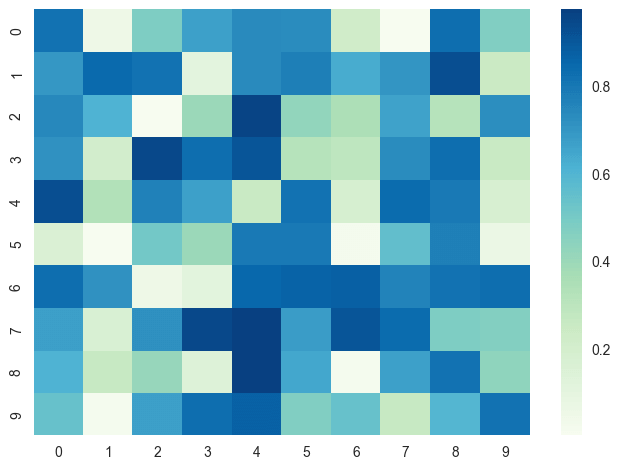SofaSofa数据科学社区DS面试题库 DS面经whanq   2018-01-20 11:32

3

seaborn是画图的库，比matplotlib.pyplot高级，易用一些。

SofaSofa数据科学社区DS面试题库 DS面经小舔哥   2018-01-13 11:34

2

import matplotlib.pyplot as plt
import numpy as np

a = np.random.random((16, 16))
plt.imshow(a, cmap='hot', interpolation='nearest')
plt.show()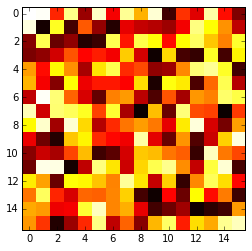SofaSofa数据科学社区DS面试题库 DS面经abuu   2018-01-19 10:25

1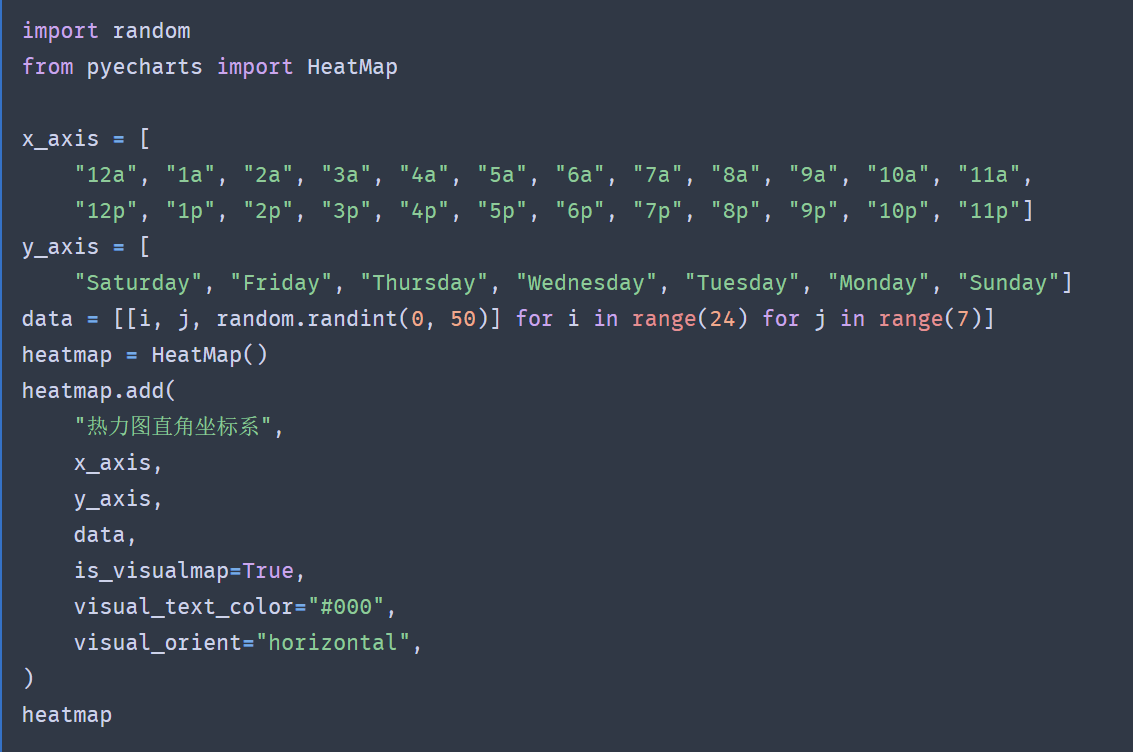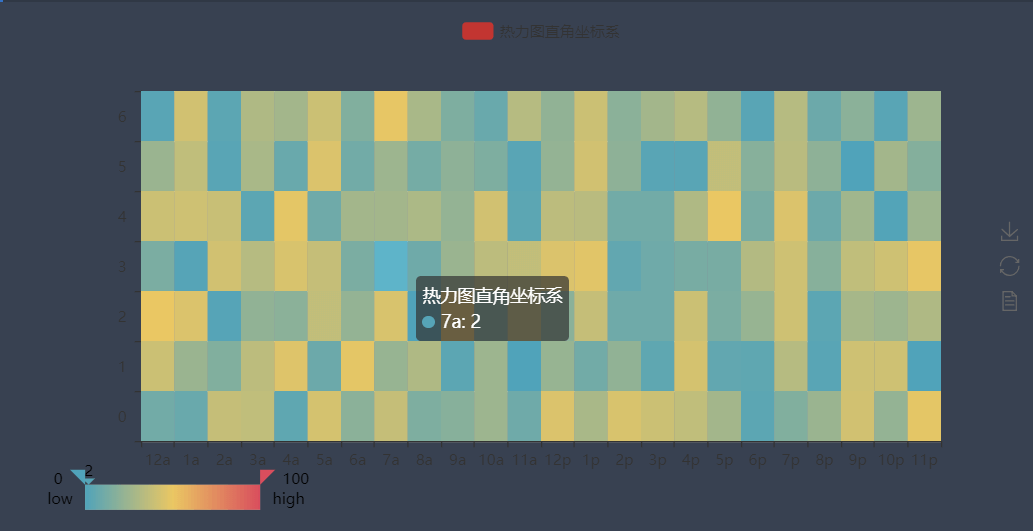pyecharts可以画出比较漂亮的图，而且是可以交互的下面是官方文档

SofaSofa数据科学社区DS面试题库 DS面经zzt5566   2018-08-31 23:14相关主题